Aegidius
Plüssin tutorials/applications
Use the asterisk (*) character for wildcard searches
Searches on more than one word will be treated "as a phrase"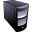Server Status
www.aplu.ch
www.java-online.eu
Print Text
© 2019, V10.1Java exemplarisch (Textbook, in German)Programming Concepts in Python Tutorial and Exercises in German, French and EnglishTCPCom An event-driven socket libarry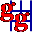JGameGrid        (Java Game Framework)JCardGame (JGameGrid Card Game Addon) Ported to AndroidJDroidLib     NEW: Support for Smartphone IDE (JGameGrid/TcpJLib for Android)JTurtleLib     (Java Turtle Graphics for Android)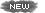Python-Exemplary Raspberry Pi Tutorial (in English and German)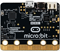New micro:bit     Single-board Computer Python Tutorial (Now released!)BrickPiLib Library for the BrickPi KitRaspiBrick Low-Budget Robotics with the Raspberry Pi EV3JLib (Library for Lego EV3 Robot) Support for Jython & AndroidTcpJLib (TCP/IP Communication)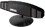KinectJLib (Kinect Controller Library for Java)JAW       (Java API Wrapper, 32/64bit)Python/Jython (Using TigerJython IDE)Java Utilities  (Graphics, Turtle)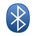Bluetooth Lib (For J2ME/J2SE)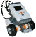NxtJLib (Library for Lego NXT Robot) Support for J2SE/J2ME & AndroidXboxController (Xbox Gamepad Library)Simulations    (for Physics Teachers)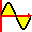Overtone           (Spectral Composition/Analysis)FtController  (Fischertechnik Java Library) CoverSheet      (Presentation Tool) Gidlet      (Java ME Framework) MidPak      (MIDlet Packaging) JavaOC      (Java Object Creator) JawGadget (Transparent Windows) C++/Champ      (Graphics Framework) Contact Home
JGameGridThe source code of all examples is included in the JGameGrid distribution.

JGameGrid's GGPanel Graphics

The JGameGrid framework is dedicated to gaming but it's frame window may also be used to display simple graphics with a friendly user definable double coordinate system similar to the GPanel class in the ch.aplu.util package. Such graphics tools are frequently used in introductory programming courses because they hides the tedious code for creating and managing the GUI window. In JGameGrid the GPanel class becomes GGPanel (with the GG prefix like most other framework classes). The documentation shows that GGPanel is a thin but comfortable layer on the Graphics2D Java API class. Animation is supported because GGPanel uses a double-buffered concept: all drawing methods actually draw into a background buffer and the whole buffer is rendered on the screen by calling refresh(). If not disabled by setRefreshEnabled(false), refresh() is automatically called by each graphics method so the drawing appears incrementally. The double-buffering also avoids flickering.

In typical situations you proceed as follows:

• Create a GameGrid instance with a one pixel grid and given horizontal and vertical size
• Select the background color different to black by calling setBgColor()
• Call show() to make the window visible
• Get a GGPanel reference by calling GameGrid's getPanel() method
• Call GGPanel's window(xmin, xmax, ymin, ymax) to define the double coordinate system (keep in mind that the parameters are coordinate system x-y spans and not the upper-left/lower-right vertex coordinates)
• Use this reference to draw into the GameGrid window

In the first example we draw (once again) the famous Moiré pattern. To prolongate the pleasure we slow down the execution by waiting a moment after drawing each line.

 import ch.aplu.jgamegrid.*; import java.awt.*; public class Moire extends GameGrid {   private GGPanel p;   public Moire()   {     super(500, 500);     setBgColor(Color.white);     show();     p = getPanel();     p.window(0, 10, 0, 10);     p.color(Color.black);     for (int i = 0; i <= 10; i++)     {       for (int k = 0; k <= 10; k++)       {         p.line(i, 0, k, 10);         delay(100);       }     }     for (int i = 0; i <= 10; i++)     {       for (int k = 0; k <= 10; k++)       {         p.line(0, i, 10, k);         delay(100);       }     }   }   public static void main(String[] args)   {     new Moire();   } }

Execute the program locally using WebStart.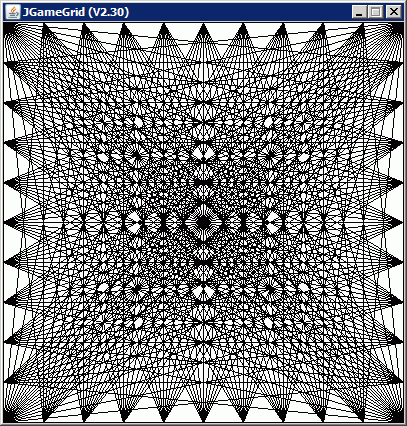The next example demonstrates how easy you draw a chessboard. Try it.

 import ch.aplu.jgamegrid.*; public class Chessboard extends GameGrid {   public Chessboard()   {     super(500, 500);     show();     GGPanel p = getPanel();     p.window(0, 8, 0, 8);     for (int i = 0; i < 8; i++)       for (int j = 0; j < 8; j++)         if ((i + j) % 2 == 0)           p.rectangle(i, j, i + 1, j + 1, true);     p.line(0, 8, 8, 8);   }   public static void main(String[] args)   {     new Chessboard();   } }

Execute the program locally using WebStart.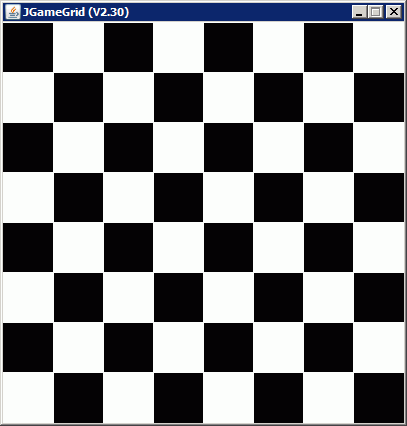Fractals are not only a subject of mathematical research but provide a great motivation and lot of fun for many computer programming courses. Fractals also enriches computer courses because of their great aesthetic appeal. Most of them use complex numbers which is unfortunately not a built-in data type in Java. So we use the Complex class of the package ch.aplu.util. The following example shows the creation of a fern leave.

 import ch.aplu.jgamegrid.*; import java.awt.*; import java.util.*; import ch.aplu.util.Complex; public class Fern extends GameGrid {   private GGPanel p;   public Fern()   {     super(400, 600);     p = getPanel();     show();     fern(20000);   }   private void fern(int nbIterations)   {     setTitle("Creating Fern...");     p.window(-3.5, 3.5, 0, 10);     Complex z = new Complex(0, 0);     int it = 0;     Random rnd = new Random();     while (it <= nbIterations)     {       delay(5);       if (it % 1000 == 0)         p.color(Color.WHITE);       double r = rnd.nextDouble();       Color color = Color.BLACK;       if (r < 0.01)       {         color = Color.ORANGE;         z = f(z, 0, 0, 0, 0.16, 0, 0); // Stem       }       else       {         if (r < 0.86)         {           color = Color.GREEN;           z = f(z, 0.85, 0.04, -0.04, 0.85, 0, 1.60); // symmetry         }         else         {           if (r >= 0.86 && r < 0.93)           {             color = Color.RED;             z = f(z, 0.20, -0.26, 0.23, 0.22, 0, 1.60); // left leaves           }           else           {             if (r >= 0.93)             {               color = Color.BLUE;               z = f(z, -0.15, 0.28, 0.26, 0.24, 0, 1.44); // right leaves             }           }         }       }       p.color(color);       p.point(z.real, z.img);       it++;     }     setTitle("Creating Fern...Done.");   }   private Complex f(Complex z,     double a, double b, double c, double d, double e, double f)   {     double re = a * z.real + b * z.img + e;     double im = c * z.real + d * z.img + f;     return new Complex(re, im);   }      public static void main(String[] args)   {     new Fern();   } }

Execute the program locally using WebStart.Another famous fractal is the Mandelbrot set where f(z) = z * z + c is iterated in every grid point of the complex plane until the magnitude exceeds a certain limit. Then the color of that particular point is drawn depending on the number of iterations. One of the fractal's property is self-similarity that is shown in the following example where a part of the fractal image can be selected by a mouse drag and is same algorithm is applied to the selected area (not to be mistaken for a simple zoom operation).

 import ch.aplu.jgamegrid.*; import ch.aplu.util.*; import java.awt.Color; import java.awt.event.*; public class Mandelbrot extends GameGrid   implements MouseListener, MouseMotionListener {   private final int maxIterations = 50;   private final double res = 800;   private GGPanel p;   private double xStart;   private double yStart;   private double xEnd;   private double yEnd;   private boolean isDrawing = false;   public Mandelbrot()   {     super(500, 500);     show();     p = getPanel();     p.setRefreshEnabled(false);     addMouseListener(this);     addMouseMotionListener(this);     draw(-2, 2, -2, 2);     while (true)     {       Monitor.putSleep();       p.clear();       refresh();       double xmin = Math.min(xStart, xEnd);       double xmax = Math.max(xStart, xEnd);       double ymin = Math.min(yStart, yEnd);       double ymax = Math.max(yStart, yEnd);       draw(xmin, xmax, ymin, ymax);     }   }   private void draw(double xmin, double xmax, double ymin, double ymax)   {     isDrawing = true;     setTitle("Working. Please wait...");     p.window(xmin, xmax, ymin, ymax);     double span = xmax - xmin;     Complex vector = new Complex(xmin, ymin);     for (double i = 0; i < res; i++)     {       for (double j = 0; j < res; j++)       {         Complex c = new Complex(span / res * i, span / res * j);         c.add(vector);         Complex z = new Complex(0, 0);         int k = 0;         while (z.modulus() < 2 && k < maxIterations)         {           // z = z * z + c           z.multiply(z);           z.add(c);           k++;         }         putPixel(c, new Color((30 * k) % 256, (4 * k) % 256,           255 - (30 * k) % 256));       }       refresh();     }     isDrawing = false;     setTitle("Done. Select zoom area!");   }   public void mousePressed(MouseEvent evt)   {     if (isDrawing)       return;     xStart = xEnd = p.toUserX(evt.getX());     yStart = yEnd = p.toUserY(evt.getY());     p.setXORMode(Color.white);   }   public void mouseDragged(MouseEvent evt)   {     if (isDrawing)       return;     p.color(Color.red);     drawRectangle(true);     xEnd = p.toUserX(evt.getX());     yEnd = p.toUserY(evt.getY());     drawRectangle(false);     refresh();   }   public void mouseReleased(MouseEvent evt)   {     if (isDrawing)       return;     drawRectangle(true);     p.setPaintMode();     refresh();     if (!(xStart == xEnd && yStart == yEnd))       Monitor.wakeUp();   }   private void drawRectangle(boolean erase)   {     if (!erase)     {       double dx = xEnd - xStart;       double dy = yEnd - yStart;       double s = Math.min(Math.abs(dx), Math.abs(dy)); // Side of square       double dxx = Math.signum(dx) * s;       double dyy = Math.signum(dy) * s;       xEnd = xStart + dxx;       yEnd = yStart + dyy;     }     if (!(xStart == xEnd && yStart == yEnd))       p.rectangle(xStart, yStart, xEnd, yEnd, false);   }   private void putPixel(Complex z, Color c)   {     p.color(c);     p.point(z.getReal(), z.getImg());   }   public void mouseEntered(MouseEvent e)   {   }   public void mouseExited(MouseEvent e)   {   }   public void mouseMoved(MouseEvent e)   {   }   public void mouseClicked(MouseEvent e)   {   }   public static void main(String[] args)   {     new Mandelbrot();   } }

Execute the program locally using WebStart.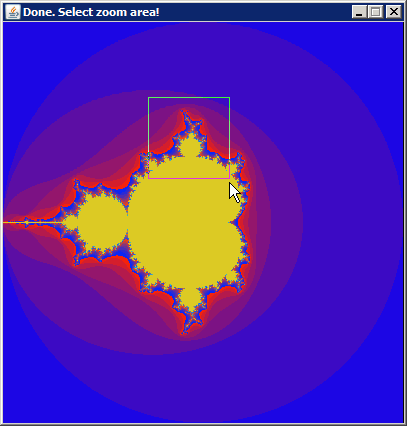Tutorial Page 1 of 16 Tutorial Page 2 of 16 Tutorial Page 3 of 16 Tutorial Page 4 of 16 Tutorial Page 5 of 16 Tutorial Page 6 of 16 Tutorial Page 7 of 16 Tutorial Page 8 of 16 Tutorial Page 9 of 16 Tutorial Page 10 of 16 Tutorial Page 11 of 16 Tutorial Page 12 of 16 Tutorial Page 13 of 16 Tutorial Page 14 of 16 Tutorial Page 15 of 16 Tutorial Page 16 of 16 Tutorial Appendix 1 Tutorial Appendix 2 Tutorial Appendix 3 Tutorial Appendix 4 Tutorial Appendix 5 Tutorial Appendix 6 Tutorial Appendix 7Applications 1 of 5 Applications 2 of 5 Applications 3 of 5 Applications 4 of 5 Applications 5 of 5 Miscellany EV3/NXT Simulation How to port JGameGridprograms to Android(and vice-versa) Free Download (includes JCardGame) V 2.47 - June 6, 2017 Download Source Demo (Implementation of basic features only) FAQ/Forum ch.aplu.jgamegrid JavaDoc JavaDoc Spiele als Einstieg in das objektorientierte Programmieren (Zeitschrift für Didaktik der Informatik 1 (2010) Einstieg in die Objekt- orientierte Programmierung mit BlueJ und JGameGrid (DUE im Fach Informatik) GameGrid Tutorial PHBern (Institute of Teacher Training) GameGridKara (English) Learning Java with Kara GameGridKara(German) Java lernen mit Kara GameGridKara Source Distribution Free Sprite Libraries: Open IconLib FlyingYogi SpriteLib Nasa ImageLib Clker ClipArt Java-Online SpriteLib GameGrid Softpedia Link: JGameGrid GameGrid Softpedia Link: Brownian Motion GameGrid SoftpediaLink: Langons's Ant JGameGrid Home